SSC  >  SSC CPO Mock Test (Quant Apt) 2

# SSC CPO Mock Test (Quant Apt) 2

Test Description

## 50 Questions MCQ Test SSC CPO & Constable - Mock Tests & Previous Year Papers | SSC CPO Mock Test (Quant Apt) 2

SSC CPO Mock Test (Quant Apt) 2 for SSC 2023 is part of SSC CPO & Constable - Mock Tests & Previous Year Papers preparation. The SSC CPO Mock Test (Quant Apt) 2 questions and answers have been prepared according to the SSC exam syllabus.The SSC CPO Mock Test (Quant Apt) 2 MCQs are made for SSC 2023 Exam. Find important definitions, questions, notes, meanings, examples, exercises, MCQs and online tests for SSC CPO Mock Test (Quant Apt) 2 below.
Solutions of SSC CPO Mock Test (Quant Apt) 2 questions in English are available as part of our SSC CPO & Constable - Mock Tests & Previous Year Papers for SSC & SSC CPO Mock Test (Quant Apt) 2 solutions in Hindi for SSC CPO & Constable - Mock Tests & Previous Year Papers course. Download more important topics, notes, lectures and mock test series for SSC Exam by signing up for free. Attempt SSC CPO Mock Test (Quant Apt) 2 | 50 questions in 30 minutes | Mock test for SSC preparation | Free important questions MCQ to study SSC CPO & Constable - Mock Tests & Previous Year Papers for SSC Exam | Download free PDF with solutions
 1 Crore+ students have signed up on EduRev. Have you?
SSC CPO Mock Test (Quant Apt) 2 - Question 1

### In a school with 600 students, the average of the boys is 12 years and that of girls is 11 years. If the average age of the school is 11 years and 9 months, then the numbers of girls in the school is

SSC CPO Mock Test (Quant Apt) 2 - Question 2

### Four of the five parts named (A),(B),(C),(D) and (E) in the following equation are exactly equal. Which of the parts is not equal to the other four? The name of that part is the answer.

Detailed Solution for SSC CPO Mock Test (Quant Apt) 2 - Question 2 All equations are resulting 2x2 - 3xy + y2
except (B) gives
2x2 - xy - y2
SSC CPO Mock Test (Quant Apt) 2 - Question 3

### x2(y - z) + y2(z-x) + z2(x - y) can be factorised as

Detailed Solution for SSC CPO Mock Test (Quant Apt) 2 - Question 3 x2 (y - z) + y2 (z - x) + z2 (x - y)
= x2y - x2z + y2z - y2x + z2x - z2y
= xy(x - y) - xz (x - y) - zy (x - y) + z2 (x - y)
= (x - y) [xy - xz - zy + z2]
= (x - y) [x(y - z) - z (y - z)]
= (x - y) (y - z) (x - z)
= - (x - y) (y - z) (z - x)
SSC CPO Mock Test (Quant Apt) 2 - Question 4

In a certain party,there was a bowl of rice for every two guests,a bowl of broth for every three of them and a bowl of meat for every four of them. If in all,there were 65 bowls of food,then how many guests were there in the party?

Detailed Solution for SSC CPO Mock Test (Quant Apt) 2 - Question 4

Let the number of rice bowls be x, number of broth bowls be y
and number of meat bowls be z
∴ x + y + z = 65 .....(1)
and 2x = 3y = 4z .....(2)
By solving both the equation we get x = 30, y = 20 and z = 15 So, number of guests = 30 × 2 = 20 × 3 = 15 × 4 = 60

SSC CPO Mock Test (Quant Apt) 2 - Question 5
The average of 50 numbers is 30. If two numbers, 35 and 40 are discarded,then the average of the remaining numbers is nearly
SSC CPO Mock Test (Quant Apt) 2 - Question 6

If the roots of the quardratic equation x²-px+q = 0 differ by unity, then​

SSC CPO Mock Test (Quant Apt) 2 - Question 7

A circular running path is 726 metres in circumference. Two men start from the same point and walk in opposite directions at the speed of 3.75 km. per hour and 4.5 km per hour respectively. Then when will they meet for first chance:

Detailed Solution for SSC CPO Mock Test (Quant Apt) 2 - Question 7

The two men walk in opposite directions @ 62.5 metres/minute and 75 metres/minute respectively
∴ They will meet for the first time after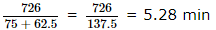SSC CPO Mock Test (Quant Apt) 2 - Question 8

The sides of a triangle are in the ratio 13:14:15 and its perimeter is 168cm. The length of the altitude corresponding to the longest side is

Detailed Solution for SSC CPO Mock Test (Quant Apt) 2 - Question 8

Ratio of sides = 13 : 14 : 15
Perimeter of triangle = 168 cm
Let the sides are 13x, 14x, and 15x
∴ 13x + 14x + 15x = 168
⇒ 42x = 168
⇒ x = 4
∴ sides are 13 × 4 = 52 cm
14 × 4 = 56 cm
15 × 4 = 60 cm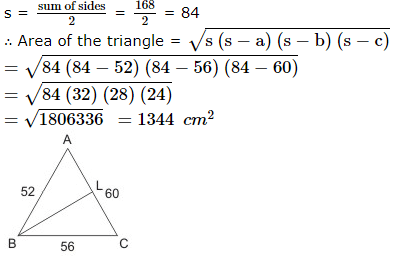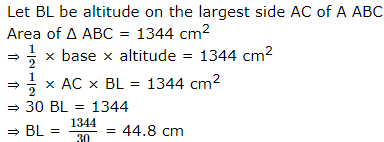SSC CPO Mock Test (Quant Apt) 2 - Question 9

The average weight of a class of 24 students is 35 kg. If the weight of the teacher be included, the average rises by 400 g. The weight of the teacher is:

Detailed Solution for SSC CPO Mock Test (Quant Apt) 2 - Question 9

Weight of the teacher = (35.4 × 25 - 35 × 24) kg = 45 kg

SSC CPO Mock Test (Quant Apt) 2 - Question 10

The equation x² - 7xy + 12y² = 0 represents

SSC CPO Mock Test (Quant Apt) 2 - Question 11

A sum of money lent out at simple interests amounts to Rs. 720 after 2 years and to Rs. 1020 after a further period of 5 years. The sum is :

Detailed Solution for SSC CPO Mock Test (Quant Apt) 2 - Question 11

Principal + SI for 2 years = Rs 720 ……(I)
Principal + SI for 7 years = Rs 1020 ……(II)
Subtracting equation (I) from (II),
S.I. for 5 years = (1020 – 720) = Rs 300
∴ S.I. for 2 years = 300 x 2/5 = Rs 120
∴ Principal = (720 – 120) = Rs 600

SSC CPO Mock Test (Quant Apt) 2 - Question 12

The value of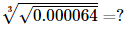SSC CPO Mock Test (Quant Apt) 2 - Question 13
After getting two successive discoubts,a shirt with a list price of Rs 150 is available at Rs 105. If the second discount is 12.5% the first discount is
SSC CPO Mock Test (Quant Apt) 2 - Question 14
About a quadrilateral it is known that all its sides are equal. The quadrilateral is certainly a
SSC CPO Mock Test (Quant Apt) 2 - Question 15
Eight people are planning to share equally the cost of a rental car. If one person withdraws from the arrangement and the others share equally the entire cost of the car, then the share of each of the remaining persons increased by
SSC CPO Mock Test (Quant Apt) 2 - Question 16

A square is inscribed in a circle of radius 10 units. The area of the circle not included in the square......(use π = 3.14)

Detailed Solution for SSC CPO Mock Test (Quant Apt) 2 - Question 16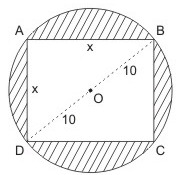Let the side of square be x unit.
sider the right triangle ABD
have x2 + x2 = (10 + 10)2
⇒ 2x2 = 400
x2 = 200
Area of square = 200 sq units
One of circle = πr2 = 22/7 x (10)2 = 314 sq units
One of shaded region not included in square
= 314 sq unit - 200 sq unit
= 114 sq unit.

SSC CPO Mock Test (Quant Apt) 2 - Question 17
Diagonals of a parallelogram are equal. The quadrilateral is a
SSC CPO Mock Test (Quant Apt) 2 - Question 18

AB∥CD and P is a point between the lines, the measure of the angles ∠ABP+∠BPD+∠CDP is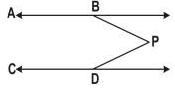SSC CPO Mock Test (Quant Apt) 2 - Question 19
Which of the following is the least perfect square which is exactly divisible by 2,3,4,5 and 6?
SSC CPO Mock Test (Quant Apt) 2 - Question 20

ABC is a triangle. ∠A = 80º, ∠C = 60º. The measure of (yº - xº) is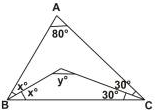Detailed Solution for SSC CPO Mock Test (Quant Apt) 2 - Question 20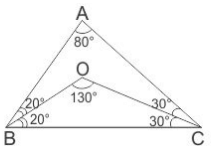∠ABC = 180º - (∠BAC + ∠ACB)
∠ABC = 180º - 140º
∠ABC = 40º
But ∠ABC = 2xº
⇒ 2x = 40º
⇒ xº = 20º
Hence,
∠BOC = 180º - (20º + 30º)
∠BOC = 130º
⇒ yº - xº = 130º - 20º
yº - xº = 110º

SSC CPO Mock Test (Quant Apt) 2 - Question 21

From a point P on a level ground, the angle of elevation of the top of a tower is 30º. If the tower is 100 m high, the distance of point P from the foot of the tower is:

SSC CPO Mock Test (Quant Apt) 2 - Question 22
If 2579*84 is divisible by 11,the missing digit is
SSC CPO Mock Test (Quant Apt) 2 - Question 23
5% income of A is equal to 15% income of B and 10% income of B is equal to 20% income of C.If the income of C is Rs 2000,then the total income of A,B and C (in Rs) is
SSC CPO Mock Test (Quant Apt) 2 - Question 24
10% of the voters did not cast their vote in an election between two candidates. 10% of the votes polled were found invalid. The successful candidate got 54% of the valid votes and won by a majority of 1620 votes. The number of voters enrolled on the voters list was:
SSC CPO Mock Test (Quant Apt) 2 - Question 25
A cistern can be filled by a tap in 4 hrs while it can be emptied by another tap in 9 hrs. If both the taps are opened simultaneously, after how much time will the cistern get filled?
SSC CPO Mock Test (Quant Apt) 2 - Question 26

A house worth Rs 150000 is sold by X at 5% profit to Y. Y sells the house back to X at a 2% loss. Then, in the entire transaction

Detailed Solution for SSC CPO Mock Test (Quant Apt) 2 - Question 26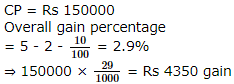SSC CPO Mock Test (Quant Apt) 2 - Question 27
At what percent above the cost price must a shopkeeper mark his goods so that he gains 20% even after giving a discount of 10% on the marked price?
SSC CPO Mock Test (Quant Apt) 2 - Question 28
If a:b=2:5,then (3a+4b):(5a+6b) is equal to
SSC CPO Mock Test (Quant Apt) 2 - Question 29

The equation ax2 + bx + c = 0, where a, b, c are the sides of a ΔABC , and the equation x2 + √2x + 1 = 0 have a common root. The measure of ∠C is​

Detailed Solution for SSC CPO Mock Test (Quant Apt) 2 - Question 29

Clearly, the roots of x2 + √2x + 1 = 0 are nonreal complex. So, one root common implies both roots are common.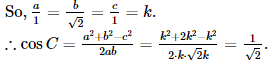SSC CPO Mock Test (Quant Apt) 2 - Question 30
An alloy contains copper and Zinc in the ratio 5:2 and another alloy containing Zinc and tin in the ratio 3:4. If two parts of first alloy and one part of second alloy are melted together to form a new alloy of copper,zinc and tin, the ratio of metals in the new alloy will be
Detailed Solution for SSC CPO Mock Test (Quant Apt) 2 - Question 30 Allay A contains Copper and Zinc in the ratio 5 : 2
Alloy B contains Zinc and tin in the ratio 3 : 4
The new alloy contains 2 parts of alloy A that means copper and zinc ratio is 10 : 4
And
One part of alloy B that means zinc and tin ratio is 3 : 4
So copper = 10
Zinc = 4 + 3 = 7
tin = 4
So the ratio of metals in the new alloy will be 10 : 7 : 4
SSC CPO Mock Test (Quant Apt) 2 - Question 31

11³ + 12³ + 13³ + ... + 20³ is

SSC CPO Mock Test (Quant Apt) 2 - Question 32
What sum of money will amount to Rs 520 in 5 yrs and to Rs 568 in 7 yrs at simple interest?
SSC CPO Mock Test (Quant Apt) 2 - Question 33
The positive square root of 45.5625 is
SSC CPO Mock Test (Quant Apt) 2 - Question 34
Walking at 3/4 of his usual pace, a man reaches his office 20 minutes late. Find his usual time.
SSC CPO Mock Test (Quant Apt) 2 - Question 35
A contractor undertakes to excute a certain work by 10 days and engages 12 men. He finds that half the work is done in 6 days. Number of additional men that he must now employ to fulfill his contract is
SSC CPO Mock Test (Quant Apt) 2 - Question 36

A circular wire of radius 7 cm is cut and bend again into an arc of a circle of radius 12 cm. The angle subtended by the are at the centre is​

SSC CPO Mock Test (Quant Apt) 2 - Question 37
The least value of cosθ+sinθ is
SSC CPO Mock Test (Quant Apt) 2 - Question 38
Cosθ/1-tanθ + sinθ/1-cotθ is equal to
SSC CPO Mock Test (Quant Apt) 2 - Question 39

A hollow spherical metallic ball has an external diameter 6 cm and is ( 1/2 ) cm thick. The volume of metal used in the ball is :

Detailed Solution for SSC CPO Mock Test (Quant Apt) 2 - Question 39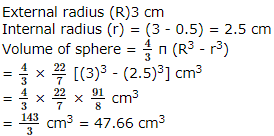SSC CPO Mock Test (Quant Apt) 2 - Question 40

The curved surface of a right circular cone of height 15 cm and base diameter 16 cm is :​

SSC CPO Mock Test (Quant Apt) 2 - Question 41

If the height of a cone can be doubled and radius of base remains the same, then the ratio of the volume of the given cone to that of the second cone will be :

Detailed Solution for SSC CPO Mock Test (Quant Apt) 2 - Question 41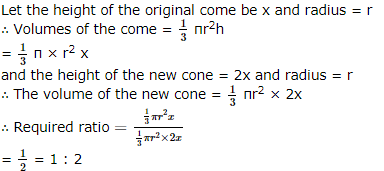SSC CPO Mock Test (Quant Apt) 2 - Question 42

Number of Vehicles Manufactured by Two companies ove the Years (Number in Thousands)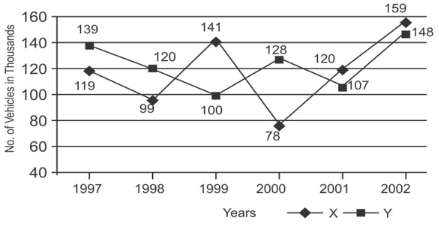Q. What is the difference between the total production of the two companies in the given years ?

Detailed Solution for SSC CPO Mock Test (Quant Apt) 2 - Question 42

Productions of Company X in the years 1997, 1998, 1999, 2000, 2001 and 2002 are 119000, 99000, 141000, 78000, 120000 and 159000 and those of Company Y are 139000, 120000,100000, 128000, 107000 and 148000 respectively.
Total production of Company X from 1997 to 2002
= 119000 + 99000 + 141000 + 78000 + 120000 + 159000 = 716000
and total production of Company Y from 1997 to 2002
= 139000 + 120000 + 100000 + 128000 + 107000 + 148000 = 742000
Difference = (742000 - 716000) = 26000

SSC CPO Mock Test (Quant Apt) 2 - Question 43

Number of Vehicles Manufactured by Two companies ove the Years (Number in Thousands)Q. What is the difference between the number of vehicles manufactured by company Y in 2000 and 2001 ?

Detailed Solution for SSC CPO Mock Test (Quant Apt) 2 - Question 43

Required difference = (128000 - 107000) = 21000.

SSC CPO Mock Test (Quant Apt) 2 - Question 44

Number of Vehicles Manufactured by Two companies ove the Years (Number in Thousands)Q. What is the average number of vehicles manufactured by company X over the given period? (rounded off to the nearest integer)

Detailed Solution for SSC CPO Mock Test (Quant Apt) 2 - Question 44

Average number of vehicles manufactured by Company X
= 1/6 × (119000 + 99000 + 141000 + 78000 + 120000 + 159000) = 119333.

SSC CPO Mock Test (Quant Apt) 2 - Question 45

Number of Vehicles Manufactured by Two companies ove the Years (Number in Thousands)Q. In which of the following years, the difference between the productions of companies X and Y was the maximum amoung the given years ?

Detailed Solution for SSC CPO Mock Test (Quant Apt) 2 - Question 45

The difference between the productions of Companies X and Y in various years are:
For 1997 (139000 - 119000) = 20000.
For 1998 (120000 - 99000) = 21000.
For 1999 (141000 - 100000) = 41000.
For 2000 (128000 - 78000) = 50000.
For 2001 (120000 - 107000) = 13000.
For 2002 (159000 - 148000) = 11000.
Maximum difference was in 2000.

SSC CPO Mock Test (Quant Apt) 2 - Question 46

These questions refer to the following circle graph showing the expenditure distribution of a certain family.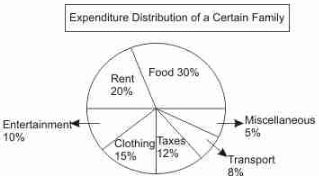Q. If the family spends Rs.6,500 per month, how much are its annual taxes ?

Detailed Solution for SSC CPO Mock Test (Quant Apt) 2 - Question 46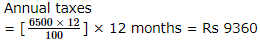SSC CPO Mock Test (Quant Apt) 2 - Question 47

These questions refer to the following circle graph showing the expenditure distribution of a certain family.Q.  How many degrees should there be in the central angle showing clothing , taxes and transportation combined ?

Detailed Solution for SSC CPO Mock Test (Quant Apt) 2 - Question 47

Required angle
= 360/100 × 35 = 126°

SSC CPO Mock Test (Quant Apt) 2 - Question 48

These questions refer to the following circle graph showing the expenditure distribution of a certain family.Q. How much more money per month is spent by the family on food as compared to the rent ?

Detailed Solution for SSC CPO Mock Test (Quant Apt) 2 - Question 48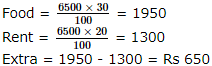SSC CPO Mock Test (Quant Apt) 2 - Question 49

These questions refer to the following circle graph showing the expenditure distribution of a certain family.Q. If the expenditure budget of the family is raised to Rs.8,000 per month and distribution on various items remain the same , then the monthly expenses on both , the entertainment and the transport, will be :

Detailed Solution for SSC CPO Mock Test (Quant Apt) 2 - Question 49

Expenses on entertainment and transport
= 8000 × 18%
= Rs 1440

SSC CPO Mock Test (Quant Apt) 2 - Question 50

The following questions are based on the following pie chart which gives the expenditure incurred in printing a magazine.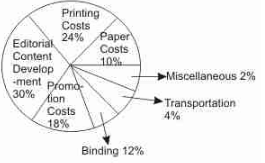Q. What is the angle for the sector representing paper cost ?

Detailed Solution for SSC CPO Mock Test (Quant Apt) 2 - Question 50

Angle for sector representing paper cost = 360/100 × 10 = 36°

## SSC CPO & Constable - Mock Tests & Previous Year Papers

1 docs|63 tests
 Use Code STAYHOME200 and get INR 200 additional OFF Use Coupon Code
Information about SSC CPO Mock Test (Quant Apt) 2 Page
In this test you can find the Exam questions for SSC CPO Mock Test (Quant Apt) 2 solved & explained in the simplest way possible. Besides giving Questions and answers for SSC CPO Mock Test (Quant Apt) 2, EduRev gives you an ample number of Online tests for practice

1 docs|63 tests